# Surface Area of Prisms and Pyramids Worksheet (with answer key+ PDF)

The shape of the base determines the surface area of a solid three-dimensional prism. A prism’s surface area is the sum of the areas occupied by its faces. Flat-faced polyhedrons are what make up prisms. It has no curves. A pyramid’s surface area is calculated by summing the areas of all of its faces. A pyramid is a three-dimensional shape whose base is a polygon and whose side faces (which are triangles) meet at what is known as the apex (or vertex).

## What is the “Surface Area of Prisms and Pyramids Worksheet”?

This worksheet will explore some of the surface areas of prisms and pyramids.

## What is the surface area of prisms and pyramids?

The total space occupied by the flat faces of a prism is referred to as its surface area. A pyramid’s surface area is a measurement of the total area occupied by all of its faces.

How will the “Surface Area of Prisms and Pyramids Worksheet” help you?

This worksheet will help the student the surface area of prisms and pyramids. Also, this worksheet will discover more about the formula, examples, and practice questions for the surface area of prisms and pyramids.

Instructions on how to use the “Surface Area of Prisms and Pyramids Worksheet.”

Examine the concept and examples provided, then attempt to complete the exercises below.

## Conclusion

A triangular prism and a triangular pyramid with equal bases and heights are related by the fact that the prism’s volume is three times that of the pyramid.

If you have any inquiries or feedback, please let us know.

## Surface Area of Prisms and Pyramids Worksheet (with answer key + PDF)

What is the Surface Area of Prisms?

The amount of space that a prism’s flat faces occupy in total is referred to as its surface area. Calculating the total area occupied by all the faces of a particular type of prism or the sum of all the surfaces in a 3D plane is how to determine a prism’s surface area.

Surface Area of a Prism Formula

The lateral surface area of the prism = base perimeter × height

The total surface area of a Prism = Lateral surface area of prism + area of the two bases = (2 × Base Area) + Lateral surface area or (2 × Base Area) + (Base perimeter × height)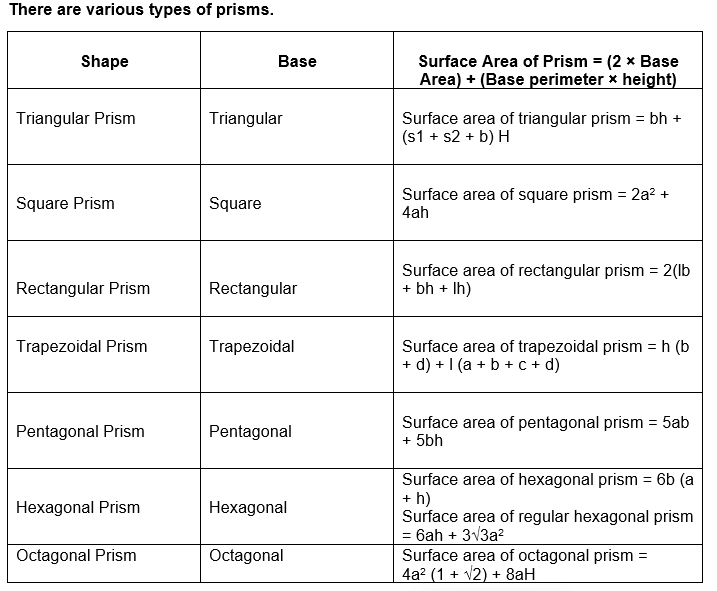How to Calculate the Surface Area of a Prism?

The steps to determine the surface area of the prism are

• Step 1: Note down the given dimensions of the prism.
• Step 2: Substitute the dimensions in the surface area of the prism formula (2 × Base Area) + (Base perimeter × height).
• Step 3: The value of the surface area of the prism is obtained and the unit of the surface area of the prism is placed in the end (in terms of square units).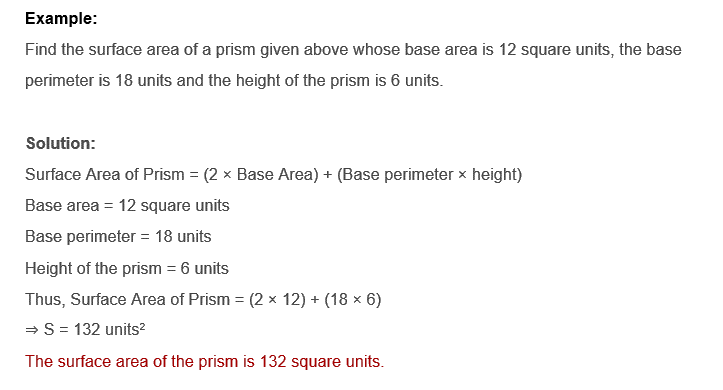What is the Surface Area of a Pyramid?

A pyramid’s surface area is a measurement of the total area occupied by all of its faces. Check out the pyramid shown below to see all of its faces as well as its apex, altitude, slant height, and base.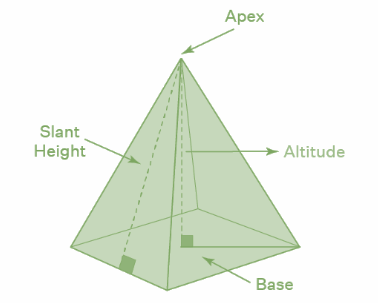A pyramid has two types of surface areas, one is the Lateral Surface Area (LSA) and the other is Total Surface Area (TSA).

• The Lateral Surface Area (LSA) of a pyramid = The sum of areas of the side faces (triangles) of the pyramid.
• The Total Surface Area (TSA) of a pyramid = LSA of pyramid + Base area

Surface Area of Pyramid Formula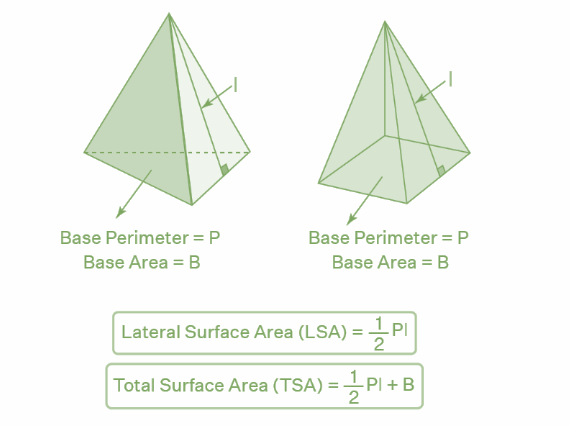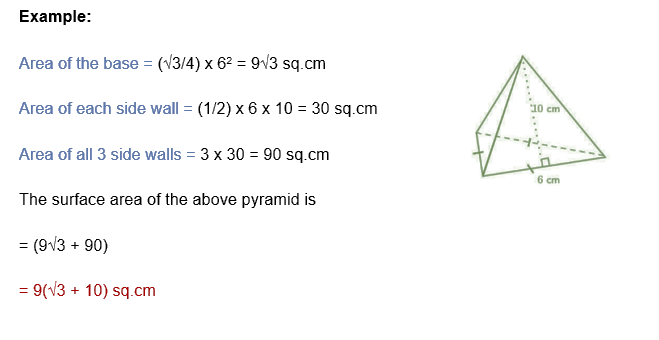## Worksheet

Solve the Surface Area of the Prisms and Pyramids.